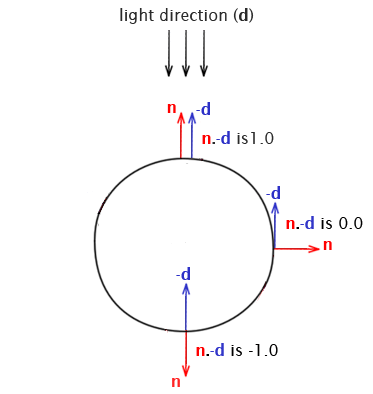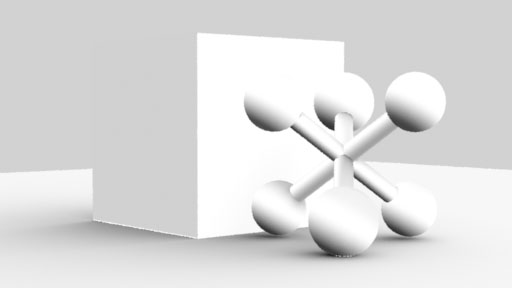### Directional Occlusion Light

#### Introduction

This tutorial develops a bi-directional "occlusionlight" shader that can be used with planar and curved surfaces. Another tutorial, " RSL: Directional Light Source Shaders", introduced a simple directional occlusion light shader - listing 1. The shader works (reasonably) well with planar surfaces that face toward the light but, as can be seen in figure 1, it fails with rear-facing surfaces.Figure 1
Rendered with shaders from listing 1 and 2.

The light source shader is unable to render the directional occlusion properly for two reasons. First, because it is implemented as a light source it calculates occlusion only for surfaces that face the light. The second reason concerns the way that most surface shaders calculate illuminance. Surface shaders typically set the sampling of their illuminance loop, or loops, to PI/2 and such hemi-spherical sampling means their rear facing surfaces do not have an opportunity to perform lighting calculations.

To render directional occlusion "properly" (what that substantively means is open to debate) requires two custom shaders ie.

• light source shader : calculates occlusion forward/backward as necessary,
• surface shader : selectively queries occlusion lights using full spherical sampling.

Listing 1 demonstrates the use of the special `__category` shader parameter to tag a light with a particular name ("occlusion"). Listing 2 shows how an illuminance loop can selectively query lights with a specific category (tag) name.

Listing 1 "occlusionlight.sl" - version 1

 ```light occlusionlight(float intensity = 1, samples = 256, multiplier = 2, coneangle = 90; color lightcolor = 1; string __category = "occlusion") { vector direction = vector "shader"(0,0,1); float occ; solar(direction, 0.0) { // The use of -direction means that only surfaces that // face the light have their occlusion calculated. occ = 1 - occlusion(Ps, -direction, samples, "coneangle", radians(coneangle)); // The multiplier darkens/lightens the occlusion. occ = pow(occ, multiplier); Cl = occ * intensity * lightcolor; } }```

Listing 2 (occlusiononly.sl)

 ```surface occlusiononly() { normal n = normalize(N); normal nf = faceforward(n, I); color accum_occlusion = 0; // Note the sampling is done over a full 360 degrees ie. PI, // not the usual hemi-spherical sampling specified by PI/2. // Also note the shader only queries lights tagged as "occlusion". illuminance("occlusion", P, nf, PI) { accum_occlusion += Cl; } Oi = Os; Ci = Oi * Cs * accum_occlusion; }```

#### Occlusion for Rear Facing Surfaces

The modified solar() statement shown below illustrates how a shader can determine the direction in which it should sample the occlusion based on the dot product (aka facing-ratio) of the direction vector of the light and the surface normal.

```    solar(direction, 0 ) {
dot = normalize(N).normalize(direction);
if(dot < 0)
direction = -direction;
occ = 1 - occlusion(Ps, direction, samples,
occ = pow(occ, multiplier);
Cl = occ * intensity * lightcolor;
}```Figure 2
Micro-polygons with a dot product less than
0.0 "face" away from the occlusion light.

Unfortunately, the new version of the occclusionlight shader, listing 3, still renders the occlusion improperly - figure 3.

Listing 3 "occlusionlight" - version 2

 ```light occlusionlight(float intensity = 1, samples = 1024, multiplier = 2, coneangle = 90; color lightcolor = 1; string __category = "occlusion") { vector direction = vector "shader"(0,0,1); float occ, dot; solar(direction, 0 ) { dot = normalize(N).normalize(direction); if(dot < 0) direction = -direction; occ = 1 - occlusion(Ps, direction, samples, "coneangle",radians(coneangle)); occ = pow(occ, multiplier); Cl = occ * intensity * lightcolor; } }```Figure 3

The abrupt darkening of the spheres is caused by 100% occlusion being applied to the micro-polygons that face away from the light - all their occlusion samples hit the floor plane. Although this is the "correct" outcome for strickly directional occlusion, aesthetically, it is objectionable because we expect the occlusion to reach a maximum value only on the parts of the spheres that more directly "face" the floor.

#### Attenuating the Sampling Direction and Cone Angle

One solution that will avoid abrupt changes in occlusion involoves biasing the sampling direction, shown as black arrows in figure 4, toward surface normal as the dot product approaches 0.0. When the dot product is 1.0 sampling occurs toward the light. When the dot product becomes -1.0, sampling occurs in the same direction as the light.

In addition to the directional sampling bias, the number of samples can also be reduced as the dot product approaches 0.0. Likewise, the value returned by the occlusion() function must also be reduced to zero when the dot product approaches 0.0.Figure 4

The final versions of the light source and surface shaders are shown in listings 4 and 5. Notice the surface shader has parameters that "control" some of the default settings of the light. This enables, for example, objects to individually set the number of occlusion sampling rays. Regrettably, there is a "fudge" factor that must be set to 0 for planar surfaces and 1 for curved surfaces. The parameter "occFudge" of the surface shader enables this factor to be set on an object by object basis.Figure 5

Listing 4 "occlusionlight.sl" - final version

 ```light occlusionlight( float intensity = 1; color lightcolor = 0; float samples = 256; float samplesMult = 1; float coneangle = 90; float coneMult = 1; float fudge = 1; string __category = "occlusion"; output varying float __occlusion = 0; ) { vector direction = vector "shader" (0, 0, 1); float samp = samples * samplesMult; float cone = coneangle * coneMult; solar(direction, 0 ) { // Reverse the direction for surfaces that face the light. float dot = normalize(N).normalize(direction); if(dot <= 0) direction = -direction; // We diminish the coneangle and fire fewer samples // as the light direction and the surface normal // approach 90 degrees ie. as the dot product becomes // closer to 0.0. if(fudge) { samp = samples * abs(dot); cone = 90.0 - degrees(acos(abs(dot))); } __occlusion = occlusion(Ps, direction, samp, "coneangle", radians(cone), "maxvariation", 0); // To avoid artifacts when the light direction and // the surface normal are near 90 degrees we fudge // the magnitude of the occlusion. The lower threshold // value of 0.05 should probably be a shader parameter. if(fudge) __occlusion *= smoothstep(0.05, 1.0, abs(dot)); __occlusion = 1 - __occlusion; Cl = intensity * lightcolor; } } ```

Listing 5 (occlusionsurface.sl)

 ```surface occlusionsurface(float Kd = 0.5, occUse = 1, /* [0 or 1] */ occFudge = 1, /* [0 or 1] */ occCone = 1, occSamples = 1; output varying float _inshadow = 0; output varying float _occlusion = 1) { normal n = normalize(N); color diffusecolor = 0, lightcolor; float accum_inshadow = 0, inshadow; float accum_occlusion = 0, occ, occ_count = 0; Oi = Os; // Query the lights of category "occlusion". Not // the use of PI rather than PI/2. illuminance("occlusion", P, n, PI, "send:light:fudge", occFudge, "send:light:coneMult", occCone, "send:light:samplesMult", occSamples ) { if(lightsource("__occlusion", occ) == 1) { occ_count += 1; accum_occlusion += occ; } } // Query the lights not of category "occlusion" illuminance("-occlusion", P, n, PI/2 ) { if(lightsource("__inshadow", inshadow) == 1) accum_inshadow += inshadow; diffusecolor += Cl * normalize(L).n; } lightcolor = diffusecolor * Kd; // Clamp the _inshadow output _inshadow = mix(0.0, 1.0, accum_inshadow); // Average the occlusion if(occ_count > 0) { _occlusion = accum_occlusion/occ_count; } // Do we wish to see the occlusion in the beauty pass? if(occUse) lightcolor *= _occlusion; Ci = Oi * Cs * lightcolor; } ```